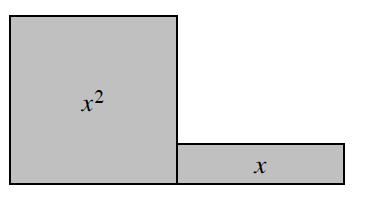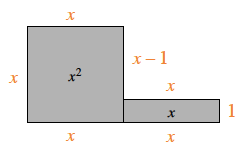### Home > CC2 > Chapter 4 > Lesson 4.3.2 > Problem4-98

4-98.

Sketch the algebra tile shape shown at right on your paper. Then:

• Write an expression for the area of the shape.

• Write and simplify an expression for the perimeter of the shape.

• Calculate the area and perimeter of the shape for each value of $x$.The area of each tile is shown in the center of it.

The perimeter is the sum of all the side lengths that do not touch another tile.

Label and add all the side lengths together:
$x+x+(x−1)+x+1+x+x$
Simplify this expression.

Add the two areas together: $x^2+x$1. $x=7$

Replace $x$ with $7$ in both expressions.

$\text{Area}=7^2+7$
$\text{Perimeter}=6(7)$

$\text{Area}=56$ sq. un.
$\text{Perimeter}=42$ un.

1. $x=2.5$

See part (a).

1. $x=15$

See part (a).